Journal of Finance and Accounting
Volume 4, Issue 3, May 2016, Pages: 86-101

Effect of Budgeting on Public Sector Wage Bill Management by the Government of Kenya

Mogere Henry Nyakundi, Simiyu Justo Masinde, Mugenda Nebat Galo

Faculty of Business Studies, Chuka University, Chuka, Kenya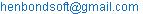(M. H. Nyakundi)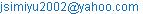(S. J. Masinde)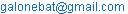(M. N. Galo)

Mogere Henry Nyakundi, Simiyu Justo Masinde, Mugenda Nebat Galo. Effect of Budgeting on Public Sector Wage Bill Management by the Government of Kenya. Journal of Finance and Accounting. Vol. 4, No. 3, 2016, pp. 86-101. doi: 10.11648/j.jfa.20160403.11

Received: March 21, 2016; Accepted: April 5, 2016; Published: May 7, 2016

Abstract: The main aim of budgeting by the government is to create plans on how to source, allocate and spend public resources prudently to meet allocation, development and stabilization objectives. All government expenditure should be controlled if both development and recurrent objectives are to be met. This is however not the case with the public sector wage bill in Kenya since it has been at a spiraling level in the recent past. The wage bill to GDP ratio was 12.1% in the year 2012/13 as compared to the internationally accepted level of 7%. The wage bill to revenue ratio was 47% in the year 2012/13 as compared to the internationally accepted levels of between 30% and 40%. The wage bill to expenditure ratio was 57% in the year 2012/13 as compared to the conventionally accepted levels of utmost 40%. This implies fiscal unsustainability of public expenditure. Public sector wage bill reforms like retrenchments, salary cuts and introduction of Salaries and Remuneration Commission to bring fiscal guidelines in wage Bill has not worked in managing the public sector wage bill in Kenya. This study aimed at assessing the effect of budgeting on public sector wage bill management in Kenya as another intervention in wage sanity. The study relied on Principal agent theory of budgeting, Top Down theory of budgeting, Bottom up theory of budgeting and incrementalism theory of budgeting which postulate that budgeting is a tool for control of expenditure. The specific objectives of the study were to establish whether the revenue forecast, recurrent expenditure budgetary projections, capital expenditure budgetary projections and expected growth in GDP have effects on public sector wage bill management. Causal research design was used to establish the cause and effect relationship between the independent and dependent variables. Purposive sampling was employed in selecting 13 fiscal year budget data. Step-wise multiple linear regression models were employed in establishing the degree and magnitude of the relationship between the variables. Student’s t-test and F-ratio were respectively applied to test hypotheses and overall significance of the regression models at 5% level of significance. The findings of this study indicates significant effect of revenue forecast, development expenditure projection and GDP on wage bill to revenue ratio and wage bill to GDP ratio and no significant effect on wage bill to recurrent expenditure ratio. It therefore implies a budget can be a control tool for expenditure. This study recommends the need for the government of Kenya to adopt program based budgeting system that factors output factor to control the wage bill.

Keywords: Wage Bill to Recurrent Expenditure, Wage Bill to Gdp Ratio, Wage Bill to Revenue Ratio, Revenue Forecast, Budget, Principal Agent Theory of Budgeting

Contents

1. Introduction

The word ‘budget’ originated from a French word ‘bougette’ meaning a little bag . In Britain, the word was used to describe the leather bag in which the chancellor of the ex-changer used to carry to the parliament, the statement of government needs and sources as described by the chancellor. After several thoughts of consensus, the budget became the document contained in the bags which represent plans of government expressed in money and submitted to legislatives for approval . The major historical function of the budget in the government was to set limits for the expenses of expenditures in order to control expenditures within those limits . Since then, all governments have been using budgets to estimate both recurrent and development expenditures for a given period along with proposals for financing them . The government carries out the budgeting exercise to plan and control resources .

The main aim of budgeting by government is to create plans on how to source, allocate and spend its resources prudently to meet its allocation, development and stabilization objectives . The budgeting process entails identifying the resources to be diverted to recurrent and development expenditure through a series of institutional approvals . A recurrent expenditure budget relates to operations of a ministry and it includes expenditures such as compensation to employees in form of salaries, stationery, transport and other related expenditures within the year.  defines development expenditure budget as investments to long term assets such as roads, schools and hospitals. According to  development and recurrent expenditure is very significant as it is based on utilization of resources to maximize their outputs, achievement of goals and creating expenditure ceilings inorder to avoid unsustainable expenditure items. , clarifies that there is a high likelyhood of inefficient and wasteful expenditure if the budget is not carefully prepared in substantial detail. This may result to inadequate funds to meet other important expenditures of the government. To that effect,  report in Kenya states that traditional budgeting system and inefficiecies may be the cause of unsustainable expenditure items like the public sector wage bill in Kenya. The internationally recognized measures of wage bill management are; wage bill to revenue ratio, wage bill to GDP ratio and wage bill to recurrent expenditure ratio. According to  the internationally acceptable level of wage bill to GDP ratio is 7%; wage bill to revenue ratio 30%-40% and wage bill to recurrent expenditure ratio not more than 40%. However, the Kenyan government has been surpassing these standard ratios raising the question as to whether the budgeting process is attaining its value of being a control mechanism to expenditure.

Public sector wage bill is an important item in all governments as it facilitates service delivery . The important role of remuneration in motivating staff confers to wage bill a status of decisive factor in improving performance . However, the public wage bill should be managed to a sustainable level if the governments are to meet current and development goals. All government resources are managed through the budget . It can therefore be noted that budgeting should influence the public sector wage bill. This has been achieved in Germany in Euro area and Japan which used the budget to manage their wage bill to GDP ratio at an average of 7% and 6.4% respectively . This could be an indicator that Euro and OECD countries are fairly managing their wage bill using the budget.

In Ghana,  notes that increases in the wage bill in the year 2001 to 2006 arose due to growth in public service employment, particularly hiring of new teachers and trade union negotiations that did not coincide with budget period. , argues that in that period, there was a serious problem in management of the public sector wage bill in Ghana since the hiring was being done arbitrarily without proper budgeting. The report further states that the budget numbers were arbitrarily scaled up without diligent consideration of emerging needs in different areas of spending. This led to problems in managing the budget if some agreements were reached at, after the budget had already been prepared. To solve this challenges, the government of Ghana in the budget statement of 2007, set up a committee to develop a framework for wage and salary administration with the goal to determine optimal number of workers to effectively and efficiently support the delivery of public services and introduction of a contingency vote to cover the anticipated cost of new pay scales . The solutions bore fruits as currently, Ghana’s wage bill to GDP ratio is averaging at 7%-8% .

In Kenya, the wage bill management has been of great concern to the government and the private sector since 1970’s . The 1960’s and 1970’s characterized massive employment of Kenyans to the civil service which rose from 14.1% to 97% with recommendation to allow civil servants to have private businesses for private incomes. The result of this is that the wage bill skyrocketed in the civil service. The period of 1980’s to 1990’s was characterized by increased pay in the civil service, government experiencing revenue shortfalls in the budget and an unsustainable civil service. The government came up with policies like reduction of mandatory retirement age from 60 years to 55 years and voluntary retirement age at 50 years. Further, the government limited government employment to 2% per annum and TSC at 5%. The hiring of temporary staff was abolished. This was in an attempt to reduce the public sector wage bill. In spite of all these efforts, the Central government wage bill as a percentage of GDP in 1990’s was about 9% . The period within 1993 to 2000 was characterized with civil service reforms like early retirement schemes, compulsory retrenchment, and focus on lenient civil service with increased productivity and scrapping of overlapping ministerial functions . The civil service reforms bore fruits as the wage bill as a percentage of GDP in the late 1990’s declined to 7% . The period of 2004 to 2007 was characterized by retirements and formation of a permanent sector pay review board with the sole role of rationalizing pay. However the wage bill was not contained.

The newly promulgated constitution of 2010 empowered introduction of The Salaries and Remuneration Commission (SRC). The Salaries and Remuneration Commission (SRC) was established under Article 230 (1) of the Constitution to bring about fiscal sustainability in wage determination . However, little has been achieved as far as wage bill sustainability is concerned. The wage bill to GDP ratio has been high in Kenya since the fiscal year 2008/09 to the fiscal year 2012/13 recording a ratio of 10.7%, 11.40%, 11.3%, 11.0% and 12.1% respectively as compared to an international figure of 7% . The wage bill to revenue ratio has been hovering between 49%-47% in the fiscal years 2008/09 to 2012/13 as compared to internationally recognized levels of between 30%-40% . The wage bill to recurrent expenditure ratio in the fiscal years 2008/09-2012/13 in Kenya was 55.2%, 54.8%, 53.0%, 55.6% and 57.0% respectively as compared to internationally recognized level of utmost 40% .

The Kenyan government through its growth blueprint as envisioned in the vision 2030 expects tremendous growth in all perspectives of life namely the social, economic and political dimensions.To achieve this goal, management and prudent use of scarce resources to lay a strong foundation for this growth is key.Overcrowding resources to a single item of expenditure may make it difficult to attain a balanced social, economic and political transformation of the country.This study is important in determining proper ways of managing the public sector wage bill so as to give room for sufficient allocation of resources to other expenditures that are also important in this transformation.

It can be noted that a lot of public sector wage bill reforms like retrenchments and reduced civil service has been done in Kenya since independence but despite all these reforms, the wage bill in Kenya remains high. There is need for further intervention to address the recurrent problem of spiraling wage bill to contain the situation. The unsustainable public sector wage bill in Kenya should be solved if the country is to develop. Therefore, this study aims at identifying the effect of budgeting on public sector wage bill management.

2. Review of Literature

2.1. Overview

The study is drawn from the budgeting theories. Organizations prepare budgets as a tool for planning and controlling organizations resources. Planning for the resources minimizes wastage of resources and facilitates its prudent use. Controlling of resources enables expenditure to be managed and put into the right use so as to realize the objectives that the organization aspires to achieve. That is why standard costing and variance analysis is carried out. Standard costing brings out the proper budgetary ceilings for an expenditure item. Variance analysis investigates the deviation from the ceilings so as to take appropriate measures to bring the expenditure into the norm. The government budget serves to promote the allocation function, the distribution function and the stabilization function . In allocation function, the budget influences the development agenda of a country through prudent allocation of funds. The distribution function serves the purpose of raising the revenue of a country through taxes and other sources of revenue. The stabilization function deals with the macroeconomic objectives that are necessary for achieving an acceptable and sustainable rate of economic growth, stable prices, including interest rates, and balance of payments. Government uses the Budget to attain and maintain a desired level of performance in the economy by ensuring that both taxes and expenditure are sustainable in the longterm . In essence therefore the budgeting theory proposes that budgeting helps in creating stability of expenditures and achieving acceptable and sustainable levels of expenditure. This applies to the public sector wage bill expenditure. The theories are as below:

2.2. Top Down Theory of Budgeting

The theory emerged in 1990’s as a result of the fiscal crisis that was encountered in budget formulation . The crisis was as a result of inefficient budget formulation in budgetary exercise in most developing countries in the world. Under this theory, the central authority or the top management places ceilings on the resources to be made available to the various expenditure items . The top down theory of budgeting advocates for efforts to be made to realize better performance, willingness to defend fiscal rules and good monitoring by the budget office, ability to prioritize programs and adherence to the rules and ceilings already prepared . The National Treasury should create rules and ceilings of wage bill expenditure so as guide on further employments and salary increases based on productivity.

2.3. Incrementalism Theory

This is a budget approach that begins with the use of the previous year budget figures as the base for the next year budget. Factors such as inflation, growths, changing condition and other information collected from forecast of the upcoming year are taken into account . The government should consider these factors before salary increases and further employments as they contribute to the performance of the economy. However, this approach may end up carrying the previous year’s inefficiency and inadequacy to the current year . Advocates of this budgeting theory argue that this approach is the most commonly used in developing countries . The disadvantages of the technique include transfer of inefficiency to the future, failure to evaluate alternatives and provision of poor information . According to  this approach fails to finance new projects of high priority sufficiently.

2.4. Principal-Agent Theory of Budgeting

According to Leruth and Paul (2007), the Principal-Agent theory of budget occurs in the public sector in various manners. It involves specifying an observable contract between the principal and the agent. In public sector, there are several Principal-Agent relationships . The examples are as follows: The Cabinet Secretary of a line ministry is the principal with the main objective of making civil servants who are the agents under him or her to implement his directives and policies. Another one can be seen between the Legislature or Parliament which acts as the principal and the Executive acting as an agent. The Parliament oversees implementation of government programs by the executive. However, according to  the relationship between the National Treasury and other line ministries is the broader form for Principal-Agent relationship in the public sector. The National Treasury acts in the capacity of the principal while supervising line ministries (agents) to ensure they produce a certain level of budget output as stated in the budget. The Accountant-General also serves in the capacity of the agent while rendering his stewardship responsibility of the government resources to the public at large. The national treasury should therefore act on improving productivity of workers in line ministries so as to create value in the cost incurred inform of salaries and wages.

3. Methodology

The nature of the study is explorative and it sought to determine the cause effect relationship that exists between budgeting and wage bill management. This required the use of causal research design which according to Frankfurt (1996) involves tests of causal relationships between variables. The design explains the changes that occur in the dependent variable as a result of the effect of the independent variables . Data was collected from The National Treasury of Kenya. The study had a target population of 21 fiscal years starting 1993/94 to 2013/14. This study employed purposive sampling which according to Kombo and Tromp (2006) is a non-probabilistic sampling technique that allows a study to use cases that have some specific characteristics with respect to the objectives of the study. The studyused 13 fiscal year budgets from the fiscal year 2000/01 to 2012/13 because of the common characteristic that the budgets within this period were prepared based on the Medium Term Expenditure Framework (MTEF) format implemented by the Kenyan government in the year 2000. A data collection checklist was employed in collecting longitudinal secondary data from the National Treasury of Kenya. Descriptive and inferential statistics was used in the analysis of data. The collected data was tested for normality using one sample kolmogorov-smirnov goodness of fit test and skewness. The data was then coded and analyzed using Statistical Package for Social Sciences (SPSS 20.0). Ordinary Least Squares method was used to determine the cause and effect relationship among the variables. Diagnostic tests including Multicollinearity, Heteroscedasticity and autocorrelation were tested to ascertain whether the assumptions of the ordinary Least Squares hold. The F- ratio was used to test the overall significance of the Estimated Multiple Linear Regression model while t-statistic was used to test the hypotheses on the individual regression coefficients at 5% level of significance. The following were the econometric models that were used:

Model 1Model 2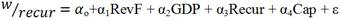Model 3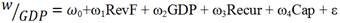Where: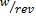is wage bill to revenue ratio for period t,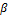1 is the fixed individual effect of revenue forecast on the wage bill to revenue ratio for period t, β0 is the constant representing the wage bill to revenue ratio when the revenue forecast, GDP, Recurrent expenditure budget and capital expenditure budget is zero, β2 is the fixed individual effect of extent of growth in GDP on the wage bill to revenue ratio for period t, β3 and β4 are the fixed individual effect of recurrent expenditure budget and capital expenditure budget on wage bill to revenue ratio for period t respectively. RevF, GDP, Recur and Cap represents revenue forecast, expected growth in GDP, recurrent expenditure budgetary projection and development expenditure budgetary projection for period t respectively. ε is the random error term which represents the effect of other factors affecting the wage bill to revenue ratio not included in the model. The error term is assumed to be normally distributed with zero mean and constant variance.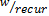is the wage bill to recurrent expenditure ratio for period t, α0 represents the constant figure of the wage bill to recurrent expenditure ratio when the revenue forecast, extent of growth in GDP, projected recurrent expenditure budget and projected capital expenditure budget is zero.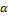1, α2, α3 and α4 are the individual effect of revenue forecast, extent of growth in GDP, projected recurrent expenditure budget and projected capital expenditure budget on the wage bill to recurrent expenditure budget for period t respectively.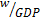represents the wage bill to GDP ratio for the period t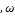0 is the constant figure of the wage bill to GDP ratio when the revenue forecast, extent growth in GDP, projected recurrent expenditure budget and projected capital expenditure budget is zero, ω1, ω2, ω3 and ω4 are the individual effect of revenue forecast, extent of growth in GDP, projected recurrent expenditure budget and projected capital expenditure budget on the wage bill to GDP ratio for period t respectively.

4. Results and Discussions

4.1. Descriptive Statistics

Table 1. Range, Mean and Standard Deviation for Model 1, 2 and 3.

 Minimum Maximum Mean Std. Deviation Capital expenditure (Ksh.millions) 38,420.8 631,692.4 194,069.793 174,138.5066 Recurrent expenditure (Ksh.millions) 264,906.7 1,001,772.2 482,414.300 210,037.6424 GDP (Ksh.millions) 882,725.0 3,797,987.8 2,032,781.485 955,429.8953 Revenue forecast (Ksh.millions) 200,377.7 971,300.0 468,745.955 256,028.1390 Wage bill to revenue ratio (%) 36.10 79.37 54.6515 15.19644 Wage bill to recurrent expenditure ratio (%) 38.88 60.25 48.5800 6.23153 Wage bill to GDP ratio (%) 8.78 15.22 11.9377 2.29692

n=13Source: Budget books and Economic survey, Fiscal years 2000/01-2012/13

In Table 1, development expenditure projections have a mean of 194,069.793 Million shillings which is an average of capital expenditure projection for the 13 fiscal year period under study. Recurrent expenditure projections have a mean of 482,414.300 Million shillings which is an average of recurrent expenditure projection for the period. GDP has a mean of 2,032,781.485 Million shillings representing an average of GDP for the period under study. Revenue forecast has a mean of 468,745.955 Million shillings representing an average of revenue forecast for the period under study. The standard deviation from the mean isshs.174, 138.5066 Million, shs.210, 037.6424 Million, shs.955, 429.8953 Million and shs.256, 028.1390 Million for capital expenditure projection, recurrent expenditure projection, expected growth in GDP and revenue forecast respectively. The mean of wage bill to revenue ratio is 54.65%. This implies that the average wage bill to revenue ratio for the 13 fiscal years has been high as compared to internationally recognized value of between 30-40%. This is consistent with the reports of  and  which states that the wage bill in Kenya has been spiraling. The dispersion from the mean is 15.19%. This implies that management of the wage bill to revenue ratio may have been difficult to be controlled within the range of 10% as suggested by IMF. The mean of wage bill to recurrent expenditure ratio is 48.58% indicating that the country faced a challenge of managing the wage bill to recurrent expenditure ratio at the utmost rate of 40% recognized internationally within the 13 years of study. The dispersion from the mean is 6.23% suggesting a challenge of maintaining a stable ratio of utmost 40%. The mean of wage bill to GDP ratio is 11.94% surpassing a recognized value of 7%internationally.Its dispersion from the mean is 2.30% meaning the country had a challenge of managing the wage bill to GDP ratio at 7%.

Table 2. Skewness of Data for Model 1, 2 and 3.

 Variable Skewness on wage bill to revenue ratio Skewness on wage bill to recurrent expenditure ratio Skewness on wage bill to GDP ratio Statistic Std. Error Statistic Std. Error Statistic Std. Error RevF .688 .616 .688 .616 .688 .616 GDP .599 .616 .599 .616 .599 .616 Recur 1.259 .616 1.259 .616 .1259 .616 Cap 1.484 .616 1.484 .616 1.484 .616

n=13

Table 2 indicates that the skewness statistic is within the range of ± 3 for wage bill to revenue ratio, wage bill to recurrent expenditure ratio and wage bill to GDP ratio which is an indication that data for model 1, 2 and 3 is normal, unbiased and it can be used reliably to make inferences in this study.

Table 3. One-Sample Kolmogorov-Smirnov Test for Model 1, 2 and 3.

 RevF GDP Recur Cap Wage bill to Recur ratio Wage bill to revenue ratio Wage bill to GDP ratio N 13 13 13 13 13 13 13 Normal Parametersa Mean 7.693 7.693 7.615 7.692 48.580 54.651 11.937 Std. Deviation 4.202 3.616 3.387 6.903 6.232 15.196 2.297 Most Absolute .151 .129 .182 .186 .203 .203 .191 Extreme Positive .151 .129 .182 .175 .203 .203 .191 Differences Negative -.147 -.114 -.158 -.186 -.143 -.143 -.180 K-Smirnov Z .543 .464 .655 .669 .854 .732 .688 Asymp. Sig. (2-tailed) .929 .982 .784 .762 .459 .658 .731

Test distribution is normal

Table 3 shows one sample kolmogorov-smirnov result for models 1, 2 and 3 which indicates that data for models 1, 2 and 3 is normal and can be used with reliability to fulfill the objectives of the study. This is because the p-values for revenue forecast, GDP, Recurrent expenditure projection, development expenditure projection, wage bill to recurrent expenditure ratio, wage bill to revenue ratio and wage bill to GDP ratio are 0.929, 0.982, 0.784, 0.762, 0.459, 0.658 and 0.731 respectively. The p-values are insignificant at 5% significance level indicating normality of the data for all models.

4.2. Heteroscedasticity Test

A residual plot was used to determine whether the dependent variables exhibit equal levels of variance across a range of independent variable(s). A regression analysis using heteroscedastic data will provide an unbiased estimate for the relationship between the dependent and independent variablesbut the standard errors will be biased causing a biased inference. Residual plot method was used to check the presence of heteroscedasticity. The residual plot revealed a near equal variance across a range of residual values.

4.3. Autocorrelation Test

Autocorrelation test was done to confirm whether an error term of one period may have a relation with the error terms for other successive periods. When the error terms of successive periods have a relation, the use of t and F statistics of significance becomes no longer valid. It therefore results to overestimated t values that may result to type 1 error. Autocorrelation is tested by use of Durbin-Watson d statistics. A value of Durbin-Watson d statistics of 0-4 indicates absence of autocorrelation.

Table 4. Autocorrelation of Data for Model 1, 2 and 3.

 Model Durbin Watson 1 1.576 2 1.224 3 1.189

The values of Durbin-Watson from Table 4 are 1.576, 1.224 and 1.189 for models 1, 2 and 3 respectively indicating absence of autocorrelation in data for all models. This is an indication that error terms in different periods have no relation and therefore the use of t and F statistics was valid in this study. This therefore improves the reliability and confidence in the inferences made.

4.4. Multicollinearity Test

The researcher tested for Multicollinearity to determine whether there is a combination of independent variables in a regression model which were highly but not perfectly correlated which might have made it difficult to isolate the effect of each independent variable on the dependent variable. This would have consequently made the standard errors for the regression coefficient estimators to become inflated which would have resulted in t-statistics becoming too small and less powerful in terms of making correct inferences. Multicollinearity is measured by Variance Inflation Factor (VIF) and tolerance. The results indicated high levels of multicollinearity of independent variables in model 1, model 2 and model 3. The researcher sought to eliminate the effect of multicollinearity in the data through stepwise regression technique which according to  involves a combination of forward selection and backward elimination of independent variables. The elimination and selection of independent variables was done so as to obtain independent variables that are not highly correlated hence eliminating multicollinearity. The stepwise regression technique resulted to dropping of some independent variables that were found to be creating high levels of multicollinearity. The regression models were therefore transformed as follows:

4.4.1. Transformed Model 1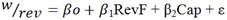Case 1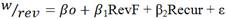Case 2

Expected growth in GDP and recurrent expenditure budgetary projection were eliminated in the first case while expected growth in GDP and capital expenditure projection were eliminated in the second case thus removing the effect of Multicollinearity with a VIF of less than 10 being obtained. The researcher adopted the use of these models in testing the null hypothesis that revenue forecast, development expenditure budgetary projection and recurrent expenditure budgetary projection have no significant effect on wage bill management for model 1.

4.4.2. Transformed Model 2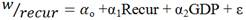Case 1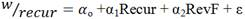Case 2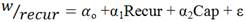Case 3

In the first case, development expenditure budgetary projection and revenue forecast were dropped from the model. In the second case, GDP and development expenditure projections were dropped from the model. In the third case, revenue forecast and GDP were dropped from the model. The result of elimination of independent variables was a VIF of less than 10. The researcher adopted the use of these models in testing the null hypothesis that recurrent expenditure budgetary projection, expected growth in GDP, revenue forecast and development expenditure budgetary projection have no significant effect on wage bill management for model 2.

4.4.3. Transformed Model 3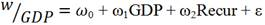Case 1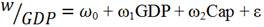Case 2

Development expenditure budgetary projection and revenue forecast were dropped from the model in case 1. Recurrent expenditure budgetary projection and revenue forecast were dropped from the model in case 2. The dropping of the independent variables resulted to elimination of Multicollinearity as the VIF was less than 10. This therefore improves the reliability and confidence in the use of these models in testing the null hypothesis that expected growth in GDP, development expenditure budgetary projection and recurrent expenditure budgetary projection for model 3.

4.4.4. Tolerance and VIF for the Transformed Models

Table 5. Tolerance and VIF for Model 1, 2 and 3.

 Tolerance VIF Model 1 RevF .0107 9.345 Case 1 Cap .0107 9.345 Model 1 RevF .136 7.340 Case 2 Recur .136 7.340 Model 2 Recur .177 5.643 Case 1 GDP .177 5.643 Model 2 Recur .155 6.457 Case 2 RevF .155 6.457 Model 2 Recur .106 9.456 Case 3 Cap .106 9.456 Model 3 GDP .112 8.901 Case 1 Recur .112 8.901 Model 3 GDP .111 9.012 Case 2 Cap .111 9.012

Dependent Variables: wage bill to revenue ratio, wage bill to recurrent expenditure and wage bill to GDP ratio.

Table 5 indicates that the transformed models have no effect of Multicollinearity since the VIF in all the models is less than 10. This improves the reliability and confidence in inferences made in this study.

4.5. Correlation

Correlation determines whether there exists a relationship between two or more variables and the magnitude of the relationship. This study applied the use of Pearson product moment correlation coefficient to determine the degree or strength of the relationship between individual variables at 5% significance level.

Table 6. Pearson Correlation Analysis for Model 1, 2 and 3.

 Model Independent variable Pearson Correlation for Wage bill to revenue ratio Pearson Correlation for wage bill to recurrent expenditure ratio Pearson Correlation for wage bill to GDP ratio Model 1 RevF -.854 Case 1 Cap -.741 Model 1 RevF -.854 Case 2 Recur -.507 Model 2 Recur -.790 Case 1 GDP -.766 Model 2 Recur -.790 Case 2 RevF -.794 Model 2 Recur -.790 Case 3 Cap -.777 Model 3 GDP -.856 Case 1 Recur -.753 Model 3 GDP -.856 Case 2 Cap -.757

Dependent variable: wage bill to revenue ratio, wage bill to recurrent expenditure ratio and wage bill to GDP ratio, n=13

The correlation between capital expenditure budgetary projection and wage bill to revenue ratio indicates a Pearson correlation coefficient of -0.741 with p-value (0.02) which is significant at 5% significance level. This suggests existence of a strong negative relationship between development expenditure budgetary projection and wage bill to revenue ratio. Capital expenditure determines the level of revenue to be raised since it focuses on infrastructural facilities. Infrastructural facilities act as a base of new revenue. The more the infrastructural facilities, the more the revenue generated. The negative relationship is therefore consistent with the findings of  which found out that the relationship is strong and negative. The Pearson correlation coefficient of wage bill to revenue ratio and revenue forecast is -0.854 with a p-value (0.000) which is significant at 5% significance level. This suggests existence of a strong negative relationship between Revenue forecast and wage bill to revenue ratio. The negative relationship is consistent with theory as proposed by IMF and quoted in the findings of  where wage bill to revenue ratio is given as total wage bill divided by the total revenue.

The Pearson correlation coefficient between wage bill to revenue ratio and recurrent expenditure budgetary projection is -0.507 with a p-value (0.001) which is significant at 5% significance level. This suggests existence of a weak negative relationship between recurrent expenditure budgetary projection and wage bill to revenue ratio. Recurrent expenditure budgetary projection has no direct influence on revenue for the period. The finding is consistent with theory by IMF and quoted in  which shows that the relationship is weak.

The Pearson correlation coefficient between wage bill to recurrent expenditure ratio and recurrent expenditure budgetary projection is -0.790 with a p-value (0.001) which is significant at 5% significance level. This suggests existence of a strong negative relationship between wage bill to recurrent expenditure ratio and recurrent expenditure budgetary projection. The findings corroborate with proposals of IMF and quoted in the findings of  which shows that wage bill to recurrent expenditure ratio is given by total wage bill divided by recurrent expenditure. The Pearson correlation coefficient between wage bill to recurrent expenditure ratio and expected growth in GDP is -0.766 with a p-value (0.001) which is significant at 95% confidence level. This suggests existence of a strong negative relationship between expected growth in GDP and wage bill to recurrent expenditure ratio. Budgetary allocations are based on GDP growths and other economic conditions like inflation. Increased GDP implies that both recurrent and development expenditure projections may increase. This finding is consistent with theory and economic reality.

The Pearson correlation coefficient between wage bill to recurrent expenditure ratio and revenue forecast is -0.794 with a p-value (0.001) which is significant at 5% significance level. This is an implication of a strong negative relationship between revenue forecast and wage bill to recurrent expenditure ratio.

The Pearson correlation coefficient between wage bill to recurrent expenditure ratio and capital expenditure budgetary projection is -0.777 with a p-value (0.001) which is significant at 5% significance level. This implies a strong negative relationship between capital expenditure budgetary projections and wage bill to recurrent expenditure ratio. Increase in capital expenditure requires proportionate increase in recurrent expenditure to support such increase. This finding is consistent with theory as proposed by IMF and in findings of  which showed a negative strong relationship.

The Pearson correlation coefficient between wage bill to GDP ratio and expected growth in GDP is -0.856 with a p-value (0.000) which is significant at 5% significance level. This is an indication of a strong negative relationship between expected growth in GDP and wage bill to GDP ratio. The finding is consistent with theory which shows that wage bill to GDP ratio is given by total wage bill divided by total GDP. The Pearson correlation coefficient between recurrent expenditure budgetary projection and wage bill to GDP ratio is -0.753 with a p-value (0.001) which is significant at 5% significance level. This suggests a strong negative relationship between recurrent expenditure and wage bill to GDP ratio. Recurrent expenditure is directly related with GDP. Recurrent expenditure is important in increasing economic activity in a country. This finding is consistent with theory and economic reality.

The Pearson correlation coefficient between capital expenditure budgetary projection and wage bill to GDP ratio is -0.757 with a p-value (0.001) which is significant at 5% significance level. This suggests a strong negative relationship between capital expenditure budgetary projection and wage bill to GDP ratio. Capital expenditure budgetary projection helps in improving the infrastructure of a country thereby contributing to growth in GDP. This finding is consistent with theory and economic reality.

4.6. Regression Analysis

The study sought to determine the causal relationship between budgeting components on public sector wage bill management. Multiple correlation coefficients (R) indicate the correlation between dependent and independent variables jointly predicted by the model. The multiple coefficient of determination (R2) determines the changes in dependent variables as explained by independent variables jointly.

4.6.1. Analysis of Model 1 Case 1

Model 1 case 1 involves wage bill to revenue ratio as a dependent variable with revenue forecast and capital expenditure budgetary projection as independent variables.

Table 7. Model 1 Case 1 Summary.

 Model R R Square Adjusted R Square Std. Error of the Estimate Durbin-Watson 1 .916a 0.838 0.806 6.00205 1.56

a. Predictors: (Constant), Cap, RevF

b. Dependent Variable: wage bill to Revenue

In Table 7, multiple coefficient of correlation (R) for model 1 case 1 was 0.916. This suggests that the degree of relation between wage bill to revenue ratio with development expenditure budgetary projections and revenue forecast is strong. The (R2) is 83.8% which means that 83.8% of the variations in the wage bill to revenue ratio can be explained by changes in revenue forecast and development expenditure budgetary projections jointly in the model and 16.2% of variation in wage bill to revenue ratio is explained by other factors not included in the model. The results indicate that the model is reliable in predicting the changes in wage bill to revenue ratio.

Table 8. Overall Significance of Model 1 Case 1.

 Model Sum of Squares Df Mean Square F Sig. 1 Regression 1869.962 2 934.981 25.954 .000a Residual 360.246 10 36.025 Total 2230.208 12

a. Predictors: (Constant), Cap, RevF

b. Dependent Variable: wage bill to Revenue

Table 8 is an ANOVA for model 1 case 1 which involves wage bill to revenue ratio as a dependent variable with revenue forecast and capital expenditure budgetary projection as independent variables. The F-test is significant at 5% level of significance (F(2,10)=25.954, p<0.05). The implication is that development expenditure budgetary projection and revenue forecast significantly explain the changes in wage bill to revenue ratio.

Table 9. Regression Coefficients for Model 1 Case 1.

 Model Unstandardized Coefficients Standardized Coefficients T Sig. B Std. Error Beta 1 (Constant) 89.895 5.726 15.700 .000 RevF -6.854 1.620 -2.113 -4.232 .002 Cap 2.571 .986 1.302 2.608 .026

Dependent Variable: wage bill to Revenue

Table 9 shows the intercept and slope coefficients for model 1 case 1. One of the aims the study sought to achieve was to determine is the effect of revenue forecast and development expenditure budgetary projections on public sector wage bill management. Table 9, shows a coefficient of -6.854 with a p-value (0.002<0.05) for revenue forecast and a coefficient of 2.571 with a p-value (0.026<0.05) for development expenditure budgetary projection. This leads to a conclusion that development expenditure budgetary projection and revenue forecast have statistically significant effect on wage bill to revenue ratio.

Model 1 Case 1 Specification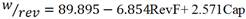(1)

According to equation 1, holding revenue forecast and development expenditure budgetary projection to be zero would result to a wage bill to revenue ratio of 89.895%. A 1% increase in revenue forecast would lead to a decrease of wage bill to revenue ratio by 6.854% and a 1% increase in development expenditure budgetary projection would lead to an increase in wage bill to revenue ratio by 2.571%. Development expenditure budgetary projection increases both the wage and the revenue. A positive coefficient of development expenditure budgetary projection implies that over the period under study, the wage bill increased more proportionately than the revenue for the period. To control the wage bill to revenue ratio, equation 1 implies that revenue should increase more proportionately than the wage bill when development expenditure and revenue forecast increases. The wage should therefore be increased in proportion to what it contributes to the revenue when revenue forecast and development expenditure budgetary projection increases. After running the regression, the expected value of the error term becomes zero and thus is not included in the model.

4.6.2. Analysis of Model 1 Case 2

Model 1 case 2 involves wage bill to revenue ratio as a dependent variable with revenue forecast and recurrent expenditure budgetary projection as independent variables.

Table 10. Model 1 Case 2 Summary.

 Model R R Square Adjusted R Square Std. Error of the Estimate Durbin-Watson 1 .886a .785 .742 6.92470 1.109

a. Predictors: (Constant), Recur, RevF

b. Dependent Variable: wage bill to Revenue

In Table 10, multiple coefficient of correlation (R) for model 1 case 2 is 0.886. This suggests that the degree of relation between wage bill to revenue ratio with recurrent expenditure budgetary projections and revenue forecast is strong. The (R2) is 78.5% which means that 78.5% of the variations in the wage bill to revenue ratio can be explained by changes in revenue forecast and recurrent expenditure budgetary projections jointly in the model. The remaining 21.5% of variation in wage bill to revenue ratio is explained by other factors not included in the model. The results suggest the model to be reliable in predicting the variations in wage bill to revenue ratio.

Table 11. Overall Significance Model 1 Case 2.

 Model Sum of Squares Df Mean Square F Sig. 1 Regression 1750.693 2 875.346 18.255 .000a Residual 479.515 10 47.952 Total 2230.208 12

a. Predictors: (Constant), Recur, RevF

b. Dependent Variable: wage bill to Revenue

Table 11 presents the results of ANOVA for model 1 case 2 which involves wage bill to revenue ratio as a dependent variable with revenue forecast and recurrent expenditure budgetary projection as independent variables. The joint contribution of recurrent expenditure budgetary projection and revenue forecast is statistically significant in explaining the changes in wage bill to revenue ratio at 5% level of significance (F(2,10)=18.255, p<0.05).

Table 12. Regression Coefficients for Model 1 Case 2.

 Model Unstandardized Coefficients Standardized Coefficients T Sig. B Std. Error Beta 1 (Constant) 72.473 5.459 13.275 .000 RevF -5.487 1.745 -1.691 -3.145 .010 Recur 3.504 2.164 .871 1.619 .136

Dependent Variable: wage bill to Revenue

The study sought to determine the effect of revenue forecast and recurrent expenditure budgetary projection on public sector wage bill management. Table 12, shows a coefficient of -5.487 with a p-value (0.010<0.05) for revenue forecast and a coefficient of 3.504 with a p-value (0.136>0.05) for recurrent expenditure budgetary projection. This leads to a conclusion that recurrent expenditure budgetary projection has a statistically insignificant effect on wage bill to revenue ratio while revenue forecast has a statistically significant effect on wage bill to revenue ratio.

Model 1 Case 2 Specification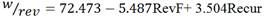(2)

Equation 2 implies that holding revenue forecast and recurrent expenditure budgetary projection to be zero would result to a wage bill to revenue ratio of 72.473%. A 1% increase in revenue forecast would lead to a decrease of wage bill to revenue ratio by 5.487% while a 1% increase in recurrent expenditure budgetary projection would result to an increase of wage bill to revenue ratio by 3.504%. According to equation 2, wage bill to revenue ratio can be controlled if the revenue increases more proportionately than the wage bill as the revenue forecast and recurrent expenditure budgetary projection increases. This can be done through program based budgeting system where the contribution of the wage to the revenue of the year is determined before further employments and salary increases rather than arbitrarily increasing the wage due to revenue forecast increases.

4.6.3. Analysis of Model 2 Case 1

Model 2 case 1 involves wage bill to recurrent expenditure ratio as a dependent variable with expected growth in GDP and recurrent expenditure budgetary projection as independent variables.

Table 13. Model 2 Case 1 Summary.

 Model R R Square Adjusted R Square Std. Error of the Estimate Durbin-Watson 1 .791a .625 .550 3.68747 1.028

a. Predictors: (Constant), GDP, Recur

b. Dependent Variable: Wage bill to Recur

In Table 13, multiple coefficients of correlation (R)for model 2 case 1 is 0.791 implying that the degree of relation between wage bill to recurrent expenditure ratio with recurrent expenditure budgetary projections and expected growth in GDP is strong. The (R2) is 62.5% suggesting that 62.5% of the changes in wage bill to recurrent expenditure ratio can be accounted by the changes in recurrent expenditure budgetary projections and expected growth in GDP jointly in the model. The remaining 37.5% of the changes in wage bill to recurrent expenditure ratio can be explained by other factors affecting the wage bill to recurrent expenditure ratio that are not included in the model. The results indicate that the changes in wage bill to recurrent expenditure ratio may not be significantly explained by the model.

Table 14. Overall significance of Model 2 Case 1.

 Model Sum of Squares Df Mean Square F Sig. 1 Regression 227.025 2 113.513 8.348 .007a Residual 135.974 10 13.597 Total 362.999 12

a. Predictors: (Constant), GDP, Recur

b. Dependent Variable: Wage bill to Recur

Table 14 is an ANOVA for model 2 case 1 which involves wage bill to recurrent expenditure ratio as a dependent variable with expected growth in GDP and recurrent expenditure budgetary projection as independent variables. The F-test is significant at 5% level of significance (F(2,10)=8.348, p<0.05). The implication of this result is that expected growth in GDP and recurrent expenditure budgetary projection significantly explains the changes in wage bill to recurrent expenditure ratio.

Table 15. Regression Coefficients for Model 2 Case 1.

 Model Unstandardized Coefficients Standardized Coefficients T Sig. B Std. Error Beta 1 (Constant) 58.301 2.603 22.395 .000 Recur -1.081 1.054 -.666 -1.026 .329 GDP -.197 .988 -.130 -.200 .846

Dependent Variable: Wage bill to Recur

Table 15 indicates the significance of the coefficients of model 2 case 1. The study sought to determine the effect of recurrent expenditure budgetary projection and expected growth in GDP on public sector wage bill management. Table 13, shows a coefficient of -1.081 with a p-value (0.329>0.05) for recurrent expenditure budgetary projection and a coefficient of -0.197 with a p-value (0.846>0.05) for expected growth in GDP. This leads to a conclusion that recurrent expenditure budgetary projection and expected growth in GDP have a statistically insignificant effect on wage bill to recurrent expenditure ratio.

Model 2 Case 1 Specification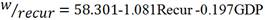(3)

Holding recurrent expenditure budgetary projection and expected growth in GDP to be zero in equation 3 would result to a wage bill to recurrent expenditure ratio of 58.301%. A 1% increase in recurrent expenditure budgetary projection would lead to a decrease of wage bill to recurrent expenditure ratio by 1.081% while a 1% increase in expected growth in GDP would result to a decrease of wage bill to recurrent expenditure ratio by 0.197%. The negative value of both the coefficients of recurrent expenditure budgetary projection and expected growth in GDP implies that the variables have a direct effect on recurrent expenditure for the period. It therefore suggests that the recurrent expenditure for the 13 fiscal year period of study increased more proportionately than the wage bill but the proportion of increase was still not substantial to reduce the wage bill to recurrent expenditure ratio at the required level. To control the wage bill to recurrent expenditure ratio, the recurrent expenditure should increase substantially than the wage expense. This can be done through adjustment of the wage based on the contribution to the economy.

4.6.4. Analysis of Model 2 Case 2

Model 2 Case 2 involves wage bill to recurrent expenditure ratio as a dependent variable with recurrent expenditure budgetary projection and revenue forecast as independent variables.

Table 16. Model 2 Case 2 Summary.

 Model R R Square Adjusted R Square Std. Error of the Estimate Durbin-Watson 1 .800a .639 .567 3.61828 1.030

a. Predictors: (Constant), RevF, Recur

b. Dependent Variable: Wage bill to Recur

In Table 16, multiple coefficients of correlation (R) for model 2 case 2 is 0.8 an implication that the degree of relation between wage bill to recurrent expenditure ratio with recurrent expenditure budgetary projections revenue forecast is strong. The (R2) is 63.9% suggesting that 63.9% of the changes in wage bill to recurrent expenditure ratio can be accounted by the changes in recurrent expenditure budgetary projections and revenue forecast jointly in the model. 36.1% of the changes in wage bill to recurrent expenditure ratio can be explained by other factors affecting the wage bill to recurrent expenditure ratio that are not included in the model. The results indicate that the model is not significant in explaining the changes in wage bill to recurrent expenditure ratio.

Table 17. Overall Significance of Model 2 Case 2.

 Model Sum of Squares Df Mean Square F Sig. 1 Regression 232.080 2 116.040 8.863 .006a Residual 130.920 10 13.092 Total 362.999 12

a. Predictors: (Constant), RevF, Recur

b. Dependent Variable: Wage bill to Recur

Statistical inference from Table 17 shows an ANOVA test for model 2 case 2 which involves wage bill to recurrent expenditure ratio as a dependent variable with revenue forecast and recurrent expenditure budgetary projection as independent variables. The F-test is significant at 5% level of significance (F(2,10)=8.863, p<0.05). This is an implication that revenue forecast and recurrent expenditure budgetary projection significantly explains the changes in wage bill to recurrent expenditure ratio.

Table 18. Regression Coefficients for Model 2 Case 2.

 Model Unstandardized Coefficients Standardized Coefficients T Sig. B Std. Error Beta 1 (Constant) 57.482 2.853 20.151 .000 Recur -.571 1.131 -.352 -.505 .624 RevF -.596 .912 -.455 -.654 .528

Dependent Variable: Wage bill to Recur

The study sought to determine the effect of recurrent expenditure budgetary projection and revenue forecast on public sector wage bill management. Table 18, shows a coefficient of -0.571 with a p-value (0.624>0.05) for recurrent expenditure budgetary projection and a coefficient of -0.596 with a p-value (0.528>0.05) for revenue forecast. This leads to a conclusion that recurrent expenditure budgetary projection and revenue forecast has a statistically insignificant effect on wage bill to recurrent expenditure ratio.

Model 2 Case 2 Specification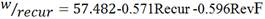(4)

According to equation 4, holding recurrent expenditure budgetary projection and revenue forecast to be zero would result to a wage bill to recurrent expenditure ratio of 57.482%. A 1% increase in recurrent expenditure budgetary projection would lead to a decrease of wage bill to recurrent expenditure ratio by 0.571% while a 1% increase in revenue forecast would result to a decrease of wage bill to recurrent expenditure ratio by 0.596%. Recurrent expenditure budgetary projection and revenue forecast directly influences the recurrent expenditure because of the negative coefficients. It is an implication that within the period of study, the recurrent expenditure increased more proportionately than the wage bill but that proportionate increase was not substantial to control the ratio at the required standards. Equation 4 suggests that to control the wage bill to recurrent expenditure ratio at standard levels, then the proportionate increase in the wage expense should be substantially lower than the recurrent expenditure when both recurrent expenditure budgetary projection and revenue forecast increases.

4.6.5. Analysis of Model 2 Case 3

Model 2 case 3 involves wage bill to recurrent expenditure ratio as a dependent variable with capital expenditure budgetary projection and recurrent expenditure budgetary projection as independent variables.

Table 19. Model 2 Case 3 Summary.

 Model R R Square Adjusted R Square Std. Error of the Estimate Durbin-Watson 1 .790a .624 .549 3.69477 1.028

a. Predictors: (Constant), Cap, Recur

b. Dependent Variable: Wage bill to Recur

In Table 19, multiple coefficients of correlation (R)for model 2 case 3 is 0.790 an implication that the degree of relation between wage bill to recurrent expenditure ratio with recurrent expenditure budgetary projections and capital expenditure budgetary projection is strong. The (R2) is 62.4% suggesting that 62.4% of the changes in wage bill to recurrent expenditure ratio can be accounted by the changes in recurrent expenditure budgetary projections and capital budgetary projection jointly in the model. 37.6% of the changes in wage bill to recurrent expenditure ratio can be explained by other factors affecting the wage bill to recurrent expenditure ratio that are not included in the model. This model is therefore not significant in affecting the wage bill to recurrent expenditure ratio.

Table 20. Overall Significance of Model 2 Case 3.

 Model Sum of Squares Df Mean Square F Sig. 1 Regression 226.486 2 113.243 8.295 .008a Residual 136.513 10 13.651 Total 362.999 12

a. Predictors: (Constant), Cap, Recur

b. Dependent Variable: Wage bill to Recur

Table 20 is an ANOVA for model 2 case 3 which involves wage bill to recurrent expenditure ratio as a dependent variable with capital expenditure budgetary projection and recurrent expenditure budgetary projectionas independent variables. The F-test is significant at 5% level of significance (F(2,10)=8.295, p<0.05).This is an implication that capital expenditure budgetary projection and recurrent expenditure budgetary projection may significantly explain the changes in wage bill to recurrent expenditure ratio.

Table 21. Regression Coefficients for Model 2 Case 3.

 Model Unstandardized Coefficients Standardized Coefficients T Sig. B Std. Error Beta 1 (Constant) 58.200 6.869 8.473 .000 Recur -1.252 1.713 -.771 -.731 .481 Cap -.015 .840 -.019 -.018 .986

Dependent Variable: Wage bill to Recur

The study sought to determine the effect of recurrent expenditure budgetary projection and development expenditure budgetary projection on public sector wage bill management. Table 21, shows a coefficient of -1.252 with a p-value (0.481>0.05) for recurrent expenditure budgetary projection and a coefficient of -0.015 with a p-value (0.986>0.05) for capital expenditure budgetary projection. This leads to a conclusion that recurrent expenditure budgetary projection and capital expenditure budgetary projection has a statistically insignificant effect on wage bill to recurrent expenditure ratio.

Model 2 Case 3 Specification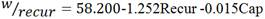(5)

Assuming recurrent expenditure budgetary projection and development expenditure budgetary projection would be zero, the wage bill to recurrent expenditure ratio would be 58.2%. A 1% increase in recurrent expenditure budgetary projection would lead to a decrease of wage bill to recurrent expenditure ratio by 1.252% while a 1% increase in development expenditure budgetary projection would result to a decrease of wage bill to recurrent expenditure ratio by 0.015%. According to equation 5, the wage bill to recurrent expenditure ratio can be controlled to required standards if the wage increases substantially less proportionately than recurrent expenditure as recurrent expenditure budgetary projection and development expenditure budgetary projection increases.

4.6.6. Analysis of Model 3 Case 1

Model 3 Case 1 involves wage bill to GDP ratio as a dependent variable with recurrent expenditure budgetary projection and expected growth in GDP as independent variables.

Table 22. Model 3 Case 1 Summary.

 Model R R Square Adjusted R Square Std. Error of the Estimate Durbin-Watson 1 .882a .778 .734 .98471 .824

a. Predictors: (Constant), Recur, GDP

b. Dependent Variable: wage bill to GDP

Table 22 indicates that the multiple coefficient of correlation (R) for model 3 case 1 is 0.882 suggesting a strong relationship between wage bill to GDP ratio with recurrent expenditure budgetary projection and expected growth of GDP. The R2 is 77.8% indicating that 77.8% of the variations in wage bill to GDP ratio are accounted by the changes in recurrent expenditure budgetary projection and expected growth in GDP jointly. 22.2% of a change in wage bill to GDP ratio is accounted for by other factors affecting the wage bill to GDP ratio that are not inclusive in the model. The results indicate that the model is significant in determining the changes in wage bill to GDP ratio.

Table 23. Overall Significance of Model 3 Case 1.

 Model Sum of Squares Df Mean Square F Sig. 1 Regression 33.996 2 16.998 17.530 .001a Residual 9.696 10 .970 Total 43.692 12

a. Predictors: (Constant), Recur, GDP

b. Dependent Variable: wage bill to GDP

Table 23 is an ANOVA for model 3 case 1 which involves wage bill to GDP ratio as a dependent variable with recurrent expenditure budgetary projection and expected growth in GDP as independent variables. The F-test is significant at 5% level of significance with a p-value of 0.001<0.05. This is an implication that recurrent expenditure budgetary projection and expected growth in GDP may significantly explain the changes in wage bill to GDP ratio.

Table 24. Regression Coefficients for Model 3 Case 1.

 Model Unstandardized Coefficients Standardized Coefficients T Sig. B Std. Error Beta 1 (Constant) 15.003 .695 21.582 .000 GDP -.814 .264 -1.543 -3.087 .011 Recur .406 .282 .720 1.441 .180

Dependent Variable: wage bill to GDP

The study sought to determine the effect of expected growth in GDP and recurrent expenditure budgetary projection on public sector wage bill management. Table 24, -shows a coefficient of -0.814 with a p-value (0.011<0.05) for expected growth in GDP and a coefficient of 0.406 with a p-value (0.180>0.05) for recurrent expenditure budgetary projection. This leads to a conclusion that recurrent expenditure budgetary projection has a statistically insignificant effect on wage bill to GDP ratio while expected growth in GDP has a statistically significant effect on wage bill to GDP ratio.

Model 3 Case 1 Specification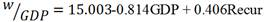(6)

The implication of equation 6 is that holding recurrent expenditure budgetary projection and expected growth in GDP to be zero would result to a wage bill to GDP ratio of 15.003%. A 1% increase in recurrent expenditure budgetary projection would lead to an increase of wage bill to GDP ratio by 0.406% while a 1% increase in expected growth in GDP would result to a decrease of wage bill to GDP ratio by 0.814%. A negative coefficient of GDP implies an inverse relationship between wage bill to GDP ratio and expected growth in GDP. An increase in expected growth in GDP results to a decrease in wage bill to GDP ratio. To control the wage bill to GDP ratio at the standard levels then, the wage expense should increase substantially less proportionately than the GDP as expected growth in GDP and recurrent expenditure budgetary projection increases.

4.6.7. Analysis of Model 3 Case 2

Model 3 Case 2 involves wage bill to GDP ratio as a dependent variable with capital expenditure budgetary projection and expected growth in GDP as independent variables.

Table 25. Model 3 Case 2 Summary.

 Model R R Square Adjusted R Square Std. Error of the Estimate Durbin-Watson 1 .886a .784 .741 .97036 1.037

a. Predictors: (Constant), Cap, GDP

b. Dependent Variable: wage bill to GDP

Table 25 indicates that the multiple coefficient of correlation (R) for model 3 case 2 is 0.886 suggesting a strong relationship between wage bill to GDP ratio with development expenditure budgetary projection and expected growth of GDP. The R2 is 78.4% indicating that 78.4% of the variations in wage bill to GDP ratio is accounted by the changes in development expenditure budgetary projection and expected growth in GDP jointly while 21.6% of changes in wage bill to GDP ratio is accounted for by other factors affecting the wage bill to GDP ratio that are not included in the model. The results indicate that the model is significant in explaining the variations in wage bill to GDP ratio.

Table 26. Overall Significance of Model 3 Case 2.

 Model Sum of Squares Df Mean Square F Sig. 1 Regression 34.276 2 17.138 18.201 .000a Residual 9.416 10 .942 Total 43.692 12

a. Predictors: (Constant), Cap, GDP

b. Dependent Variable: wage bill to GDP

Table 26 is an ANOVA for model 3 Case 2 which involves wage bill to GDP ratio as a dependent variable with capital expenditure budgetary projection and expected growth in GDP as independent variables. The F-test is significant at 5% level of significance with a p-value of 0.000<0.05. This is an implication that capital expenditure budgetary projection and expected growth in GDP may significantly explain the changes in wage bill to GDP ratio.

Table 27. Regression Coefficients for Model 3 Case 2.

 Model Unstandardized Coefficients Standardized Coefficients T Sig. B Std. Error Beta 1 (Constant) 16.743 1.132 14.794 .000 GDP -.864 .275 -1.637 -3.137 .011 Cap .225 .144 .815 1.561 .150

Dependent Variable: wage bill to GDP

The study sought to determine the effect of expected growth in GDP and development expenditure budgetary projection on public sector wage bill management. Table 27, shows a coefficient of -0.864 with a p-value (0.011<0.05) for expected growth in GDP and a coefficient of 0.225 with a p-value (0.150>0.05) for development expenditure budgetary projection. This leads to a conclusion that development expenditure budgetary projection has a statistically insignificant effect on wage bill to GDP ratio while expected growth in GDP has a statistically significant effect on wage bill to GDP ratio.

Model 3 Case 2 Specification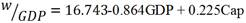(7)

According to equation 7, holding development expenditure budgetary projection and expected growth in GDP to be zero would result to a wage bill to GDP ratio of 16.743%. A 1% increase in development expenditure budgetary projection would lead to an increase of wage bill to GDP ratio by 0.225% while a 1% increase in expected growth in GDP would result to a decrease of wage bill to GDP ratio by 0.864%. A negative coefficient of GDP implies an inverse relationship between wage bill to GDP and expected growth on GDP while a positive coefficient of development expenditure budgetary projection shows a direct relationship between wage bill to GDP ratio and development expenditure budgetary projection. The wage bill to GDP ratio can be controlled at standard levels if the wage expense is made to increase substantially less proportionately than the GDP when the expected growth in GDP and development expenditure budgetary projection increases.

5. Findings and Conclusions

The study found out that revenue forecast and development expenditure budgetary projection have significant effect on wage bill to revenue ratio while recurrent expenditure budgetary projection has no significant effect on wage bill to revenue ratio. This led to a conclusion that a more than proportionate increase in revenue forecast and development expenditure budgetary projection as compared to the public sector wage bill would result to a decrease in wage bill to revenue ratio and therefore maintaining it at recognized levels. It was also found out that recurrent expenditure budgetary projection, GDP, revenue forecast and development expenditure budgetary projection have no significant effect on wage bill to recurrent expenditure ratio. This led to a conclusion that public sector wage bill should be effectively factored in the budget to control wage bill to recurrent expenditure ratio and hence the proportionate increase in recurrent expenditure budgetary projection, expected growth in GDP, revenue forecast and development expenditure budgetary projection should be more than the public sector wage bill to control wage bill to recurrent expenditure ratio. Further, expected growth in GDP was found to have a significant effect on wage bill to GDP ratio, while recurrent expenditure budgetary projection and development expenditure budgetary projection were found to have no significant effect on wage bill to GDP ratio. This led to a conclusion that there should be a more than proportionate increase in expected growth in GDP as compared to the public sector wage bill to control wage bill to GDP ratio.

In general, budgets if utilized properly can act as a control tool in managing wage bill expenditure in Kenya as advanced in Bottom up theory of budgeting, Top Down theory of budgeting, incrementalism theory of budgeting and Principal-Agent theory of budgeting which advocates that budgets act as ceilings and control tools in expenditure items. This can be done through consideration of output factor before further increment of salaries and further employments are done.

References

1. Abdullahi, A. M., & Angus, O. U. (2012). Budget in Nigerian Public Sector: Need for Balanced Scorecard Perspective.International Journal of Finance and Accounting 2012, 1(2): 1-6 DOI: 10.5923/j.ijfa.20120102.01, 1-5.
2. Aczel, A., & Sounderpadian, J. (2002). Business Statistics fifth edition. New York: Irwin: McGraw hill companies.
3. Adebayo, O., Lawrence, I., & Sola, A. T. (2013). Budgetary Control: A Tool for Cost Control in Manufacturing Companies in Nigeria. International Journal of Innovative Research and Studies 2(12), 689-710.
4. Aliu, O. A., Salam, M. O., & Abdulkadir, R. I. (2009). An Assessment of Influence Of Budget Process on Budget Perfomance:The expperience of Kwara State. Academy of Taiwan Business Management Review, 85-92.
5. Bammeke, S. (2008). Public Sector Accounting and Finance for Decision Making.Lagos: SAB and Associate.
6. Centre for Policy Analysis. (2007). Issues in Public Expenditure Management in Ghana: 2001-2006. Accra: Centre forPolicy Analysis No. 11 Amilcar Carbral Road Airport.
7. Cowen, S., & Dean, B. (1979). The Use of Zero-based Budgeting in Local Government: Some Observations. Interfaces 9(4), 61-66.
8. Dandago, K., & Tijani, B. (2003). Cost and Management Accounting. Lagos: Malthouse.
9. Edward, J., Kung, H., Gary, C., & Thomas, W. (2005). Cost Management: A Strategic Emphasis.NewYork:McGraw-Hill Irwin.
10. Frankfort, N. (1996). Research Methods in the Social sciences. London: St. Martin's Press, Inc.
11. Holm-Hadulla, F., Kamath, K., Lamo, A., Perez, J., & Schuknecht, L. (2010). Public Wages in the Euro area, Towards Securing Stability and Competitiveness. Occassional Paper Series no 112, European Central Bank.
12. Institute of Economic Affairs. (2012). Citizens hand book on budget second ediition, a guide to the budget process in Kenya pp 1-72. Nairobi: Government printers.
13. Institute Of Economic Affairs. (2007). The Citizen's Handbook on the Budget secondedition. Nairobi: Institute Of Economic Affairs.
14. Kipkirui, G. C. (2009). Analysis of the Budgetary process in Kenya and recommendations for improvement. Published Report. KDI school of public policy and management.
15. Kombo, D., & Tromp, D. (2006). Proposal and Thesis Writting. Nairobi: Paulines Publications Africa.
16. Kothari, C. (2004). Research Methodology: Methods and Techniques, second revised edition. New Delhi: New Age International (P) Ltd., Publishers.
17. Leruth, L., & Paul, E. (2007). "A Principal-Agent Approach to Public Expenditure Management in Developing Countries." OECD Journal on Budgeting, 7(3), 1-30.
18. Lienert, I., & Modi, J. (1997). A Decade of Civil Service Reform in Sub-saharan Africa: IMF Working Paper WP/97/197. IMF Fiscal Affairs Department.
19. Mitchell, D. J. (2005). The impact of Government spending on economic growth.
20. Parliamentary Budget Office of Kenya. (2013). The Public Sector Wage Bill And Its implications on Economic Perfomance in Kenya. Policy Working Paper series NO 1 of 2013. Nairobi: Government Printers.
21. Pierrre, C., & Carcillo, S. (2012). Can Public Sector Wage Bill Be Reduced? National Bureau of Economic Research.
22. Poverty Reduction and Economic unit, Africa Region. (August 11, 2010). Managing Government Wage Bill for Sustained Recovery. Harare: Zimbabwe Public Expenditure Notes.
23. Republic of Kenya. (2010). The Kenyan Constitution. Nairobi: Government Printers.
24. Republic of Kenya. (2012c). The Public Finance Management Act. Nairobi: Government printer.
25. Campbell, R., & Stanley, L. (2005). Economics. New York: McGraw Hill/ Irwin.
26. Sikka, T. (2012). Fundamentals of Cost Accounting, Seventh edition. New Delhi, India: Vinod Vasishta.
27. Salaries and Remuneration Commission. (2013). Wage bill sustainability: what options for Kenya. A report issued by SRC to contain the public sector wage bill in kenya pp 1-20. Nairobi: Government Printers.

 Contents 1. 2. 2.1. 2.2. 2.3. 2.4. 3. 4. 4.1. 4.2. 4.3. 4.4. 4.5. 4.6. 5.
Article ToolsAbstractPDF(362K)